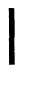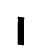Custom SearchPAR   63-65 CHAPTER   4 TROUBLESHOOTING Section   I.   GENERAL 6 3 .    P U R P O SE e:    Information   in   this   chapter i s    f o r    u s e    b y    O r d n a n c e    m a i n t e- n a n c e    p e r s o n n e l ,    i n    c o n j u n c t i o n with   and   as   a   supplement   to   the troubleshooting   section   in   the   ap- plicable   TM-20   manual.   It   provides continuation    of    instructions    where a   remedy   in   the   Unit   level   mainte- nance   manual   refers   to   Ordnance m a i n t e n a n c e    p e r s o n n e l    f o r    c o r- rective   action. Operation   of   a   deadlined   vehicle   without a   preliminary   examination   can   cause   fur- ther   damage   to   a   disabled   component   and possible   injury   to   personnel.   By   careful inspection   and   troubleshooting,   such   dam- age   and   injury   can   be   avoided.   In   addition, the   causes   of   faulty   operation   of   a   vehicle o r    c o m p o n e n t    c a n    o f t e n    b e    d e t e r m i n ed without   extensive   disassembly. 64.   GENERAL   INSTRUCTIONS   AND P R O C E D U R E S Note: This chapter contains inspection a nd troubleshooting procedures   to   be   performed   while a    d i s a b l e d    c o m p o n e n t    i s    s t i ll mounted   in   the   vehicle   as   well   as after   it   has   been   removed. .   T h e    i n s p e c t i o n s    m a d e    w h i l e    t he component   is   mounted   in   the   vehicle   are, for   the   most   part,   visual   and   are   to   be performed    before    attempting    to    operate the   vehicle.   The   object   of   these   inspec- t i o n s    i s    t o    d e t e r m i n e    t h e    c o n d i t i o n    of these   components   and,   if   found   defective, to   take   precautions   to   prevent   any   further damage. .   T h e    t r o u b l e s h o o t i n g    p e r f o r m e d while   the   component   is   mounted   in   the vehicle   is   that   which   is   beyond   the   scope o f    t h e    u s i n g    o r g a n i z a t i o n. C h e c k    t he troubleshooting   section   of   the   applicable TM-20   manual,   then   proceed   as   outlined in   this   chapter. .   If   the   component   alone   is   received by   the   Ordnance   establishment,   inspection is   performed   to   verify   the   diagnosis   made when   the   component   was   in   the   vehicle,   to uncover   further   defects,   or   to   determine malfunctions.   This   inspection   is   particu- l a r l y    i m p o r t a n t    b e c a u s e    i t    i s    o f t e n    t he only   means   of   determining   the   malfunction w i t h o u t    c o m p l e t e l y    d i s a s s e m b l i n g    t h e component. Section   II.   TROUBLESHOOTING   PROCEDURES 65.    OPERATING    CHARACTERISTICS .      .    R e f e r    to p a r s .    8    t h r o u g h    2 7    f o r    d e s c r i p t i o n    a n d T o    p e r f o r m    e f f e c t i v e    t r o u b l e s h o o t- operation   of   transmission   components. ing,   the   mechanic   must   have   a   thorough k n o w l e d g e    o f    t h e    c o n s t r u c t i o n    f e a t u r es .         .    R e f er a n d    o p e r a t i n g    c h a r a c t e r i s t i c s of the to   pars.   28   through   58   for   explanations   of power   train.   Particular   attention   should p o w e r    t r a i n    h y d r a u l i c    o p e r a t i o n s    a nd b e    a c c o r d e d    t o    t h e    s u b j e c t s    o u t l i n e d power   flow   through   the   power   train   com- b  e  l  o  w  . ponents. Change   2   69